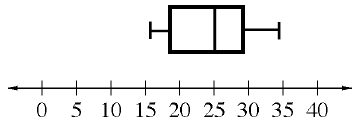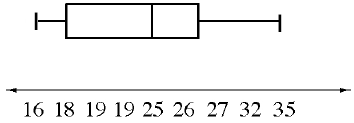### Home > MC2 > Chapter 4 > Lesson 4.2.3 > Problem4-56

4-56.

Lucy and Marissa each designed a box-and-whisker plot to represent this data set:

$16$ $18$ $19$ $19$ $25$ $26$ $27$ $32$ $35$Their plots are shown below. Which plot is scaled correctly and why? Explain the mistakes in the incorrect plot.

1.1.What are the characteristics of a number line?

A number line should be spaced evenly with numbers that increase from left to right.

Part (a) is correct because the number line is equally spaced with numbers that increase by $5$.

Part (b) is incorrect. There are two number $19$'s. In some places the numbers increase by $1$ while others increase by $3$, etc.